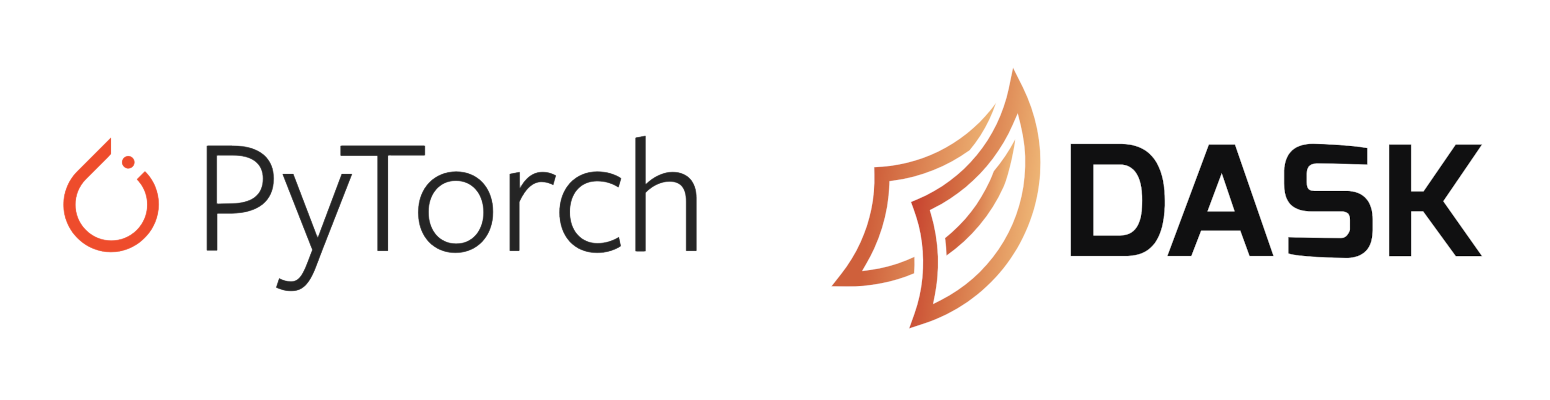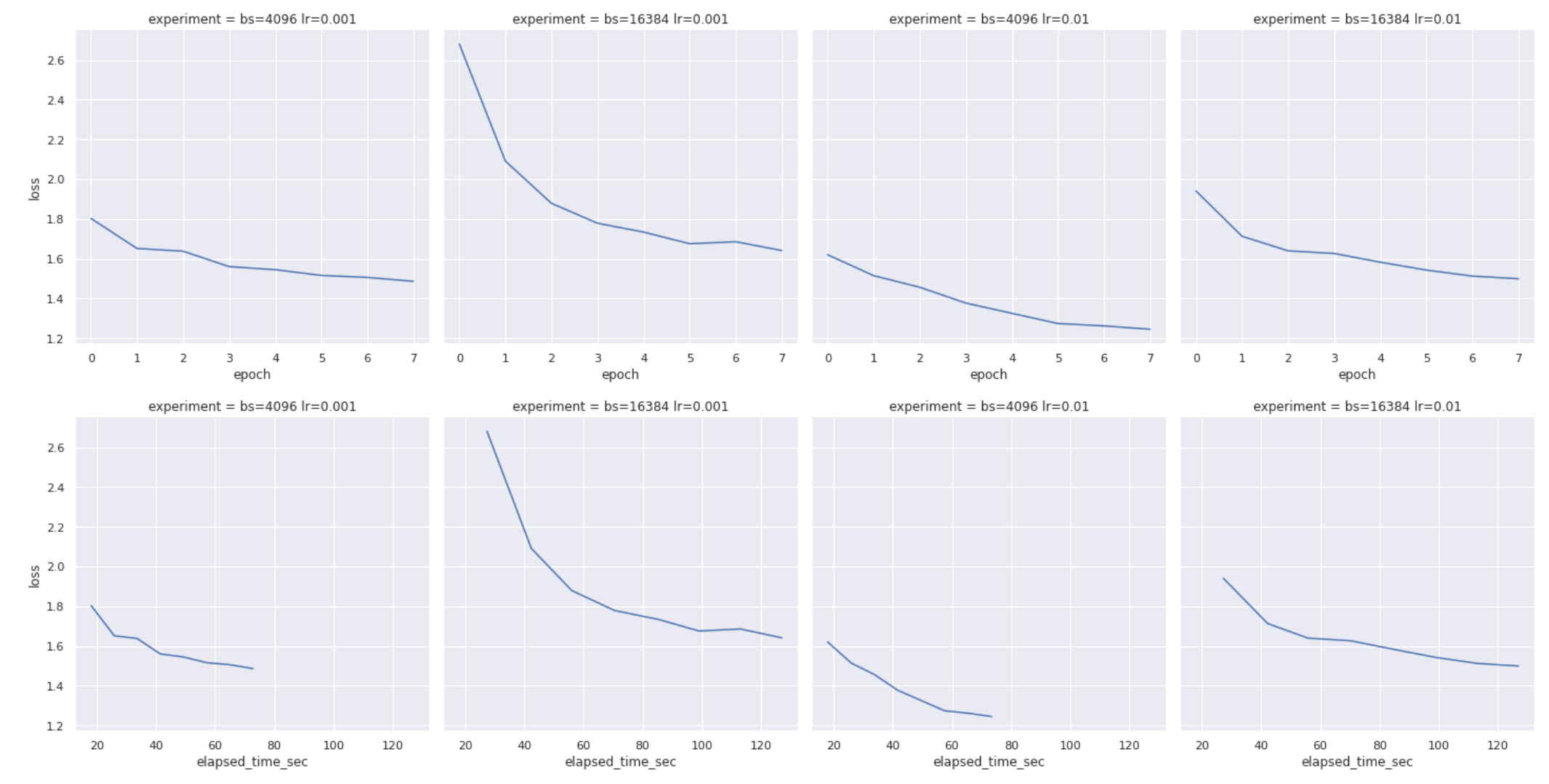# Training Many PyTorch Models Concurrently with Dask

Train multiple models with at the same time with DaskTry this example in seconds on Saturn Cloud

## Overview

This example shows how to train multiple neural networks in parallel using Dask. This is valuable for situations such as when you want to compare different parameters for a model to see which performs better. By having a cluster of machines each training a model, you can train all of the models more quickly.

This example builds on the introduction to PyTorch with GPU on Saturn Cloud example that trains a neural network to generate pet names, but instead of training the model once it trains it several times with different parameters and compares the results. Rather than having to wait for each of the different set of parameters to train sequentially, it uses the Dask cluster to train them all concurrently, dramatically speeding up the process. The code to set up the data and model architecture is the same as the original getting started with PyTorch example.

The model uses LSTM layers which are especially good at discovering patterns in sequences like text. The model takes a partially complete name and determines the probability of each possible next character in the name. Characters are randomly sampled from this distribution and added to the partial name until a stop character is generated and full name has been created. For more detail about the network design and use case, see our Saturn Cloud blog post which uses the same network architecture.

## Model training

### Imports

This code uses PyTorch and Dask together, and thus both libraries have to be imported. In addition, the `dask_saturn` package provides methods to work with a Saturn Cloud dask cluster, and `dask_pytorch_ddp` provides helpers when training a PyTorch model on Dask.

``````import datetime
import json
import torch
import torch.nn as nn
import torch.optim as optim
import urllib.request
import pandas as pd

import seaborn as sns
from distributed.worker import logger
``````

### Preparing data

This code is used to get the data in the proper format in an easy to use class.

``````with urllib.request.urlopen(
) as f:

# Our list of characters, where * represents blank and + represents stop
characters = list("*+abcdefghijklmnopqrstuvwxyz-. ")
str_len = 8
``````

Next, create a function that will take the pet names and turn them into the formatted tensors. The Saturn Cloud blog post goes into more detail on the logic behind how to format the data.

``````def format_training_data(pet_names, device=None):
def get_substrings(in_str):
# add the stop character to the end of the name, then generate all the partial names
in_str = in_str + "+"
res = [in_str[0:j] for j in range(1, len(in_str) + 1)]
return res

pet_names_expanded = [get_substrings(name) for name in pet_names]
pet_names_expanded = [item for sublist in pet_names_expanded for item in sublist]
pet_names_characters = [list(name) for name in pet_names_expanded]
pet_names_padded = [name[-(str_len + 1) :] for name in pet_names_characters]
list((str_len + 1 - len(characters)) * "*") + characters for characters in pet_names_padded
]
pet_names_numeric = [[characters.index(char) for char in name] for name in pet_names_padded]

# the final x and y data to use for training the model. Note that the x data needs to be one-hot encoded
if device is None:
y = torch.tensor([name[1:] for name in pet_names_numeric])
x = torch.tensor([name[:-1] for name in pet_names_numeric])
else:
y = torch.tensor([name[1:] for name in pet_names_numeric], device=device)
x = torch.tensor([name[:-1] for name in pet_names_numeric], device=device)
x = torch.nn.functional.one_hot(x, num_classes=len(characters)).float()
return x, y
``````

Finally, create a PyTorch data class to manage the dataset:

``````class OurDataset(Dataset):
def __init__(self, pet_names, device=None):
self.x, self.y = format_training_data(pet_names, device)
self.permute()

def __getitem__(self, idx):
idx = self.permutation[idx]
return self.x[idx], self.y[idx]

def __len__(self):
return len(self.x)

def permute(self):
self.permutation = torch.randperm(len(self.x))
``````

### Define the model architecture

This class defines the LSTM structure that the neural network will use;

``````class Model(nn.Module):
def __init__(self):
super(Model, self).__init__()
self.lstm_size = 128
self.lstm = nn.LSTM(
input_size=len(characters),
hidden_size=self.lstm_size,
num_layers=4,
batch_first=True,
dropout=0.1,
)
self.fc = nn.Linear(self.lstm_size, len(characters))

def forward(self, x):
output, state = self.lstm(x)
logits = self.fc(output)
return logits
``````

### Training multiple models in parallel

Below is the code to train the model multiple times concurrently in a distributed way using Dask. The code will start the Dask cluster connected to the Jupyter server Saturn Cloud resource, and wait for the right number of workers to be ready. You can make it take less time by starting the cluster via the UI.

``````n_workers = 3
cluster = SaturnCluster(n_workers=n_workers)
client = Client(cluster)
client.wait_for_workers(n_workers)
``````

The code to run the model multiple times in parallel starts with a training function that is extremely similar to the training function without Dask. The changes are:

• The function has a @dask.delayed indicator at the top so Dask knows to parallelize it
• The training function now has an input parameter `experiment` which is a tuple containing the batch size and learning rate
• Instead of printing the results, we now pass them to `logger.info()` so that they show up in the Dask logs.
• Instead of saving the actual model, we create an array of results that the model returns
``````@dask.delayed
def train(experiment):
num_epochs = 8
batch_size, lr = experiment
training_start_time = datetime.datetime.now()
device = torch.device(0)

dataset = OurDataset(pet_names, device=device)

model = Model()
model = model.to(device)

criterion = nn.CrossEntropyLoss()

results = []

for epoch in range(num_epochs):
dataset.permute()
for i, (batch_x, batch_y) in enumerate(loader):
batch_y_pred = model(batch_x)

loss = criterion(batch_y_pred.transpose(1, 2), batch_y)
loss.backward()
optimizer.step()
logger.info(
f"{datetime.datetime.now().isoformat()} - batch {i} - batch_size {batch_size} - lr {lr} - epoch {epoch} complete - loss {loss.item()}"
)
new_results = {
"batch_size": batch_size,
"lr": lr,
"epoch": epoch,
"loss": loss.item(),
"elapsed_time_sec": (datetime.datetime.now() - training_start_time).total_seconds(),
}
results.append(new_results)
return results
``````

### Running the parallel code

The code is executed across multiple machines by running the cell below. It takes the list of (batch size, learning rate) tuples and passes them to the Dask map function, that get combined into a single result with gather and compute. Since Dask is lazy, the computation doesn’t actually begin until you run the last line of the cell and request the results. You won’t see any messages show up as the model is being trained since all of the output is captured in the Dask logs.

``````inputs = [(4096, 0.001), (16384, 0.001), (4096, 0.01), (16384, 0.01)]

train_future = client.map(train, inputs)
futures_gathered = client.gather(train_future)
futures_computed = client.compute(futures_gathered)
results = [x.result() for x in futures_computed]
``````

### Viewing the results

This last code chunk generates several Seaborn plots of the results of the experiment. With this particular experiment the smallest batch size with highest learning rate quickly convered on a solution with fewer epochs than the other sets of parameters.

``````results_concatenated = [item for sublist in results for item in sublist]
results_df = pd.DataFrame.from_dict(results_concatenated)
results_df["experiment"] = (
"bs=" + results_df["batch_size"].astype(str) + " lr=" + results_df["lr"].astype(str)
)

sns.set_theme()

sns.relplot(data=results_df, x="epoch", y="loss", col="experiment", kind="line")

sns.relplot(data=results_df, x="elapsed_time_sec", y="loss", col="experiment", kind="line")
``````## Conclusion

With this you can now train many PyTorch GPU neural networks at the same time using Dask on Saturn Cloud. If you want to use a Dask cluster to instead train a single neural network across all of the workers, see our related example.

``````import datetime
import json
import torch
import torch.nn as nn
import torch.optim as optim
import urllib.request
import pandas as pd

import seaborn as sns
from distributed.worker import logger

with urllib.request.urlopen(
) as f:

# Our list of characters, where * represents blank and + represents stop
characters = list("*+abcdefghijklmnopqrstuvwxyz-. ")
str_len = 8

def format_training_data(pet_names, device=None):
def get_substrings(in_str):
# add the stop character to the end of the name, then generate all the partial names
in_str = in_str + "+"
res = [in_str[0:j] for j in range(1, len(in_str) + 1)]
return res

pet_names_expanded = [get_substrings(name) for name in pet_names]
pet_names_expanded = [item for sublist in pet_names_expanded for item in sublist]
pet_names_characters = [list(name) for name in pet_names_expanded]
pet_names_padded = [name[-(str_len + 1) :] for name in pet_names_characters]
list((str_len + 1 - len(characters)) * "*") + characters for characters in pet_names_padded
]
pet_names_numeric = [[characters.index(char) for char in name] for name in pet_names_padded]

# the final x and y data to use for training the model. Note that the x data needs to be one-hot encoded
if device is None:
y = torch.tensor([name[1:] for name in pet_names_numeric])
x = torch.tensor([name[:-1] for name in pet_names_numeric])
else:
y = torch.tensor([name[1:] for name in pet_names_numeric], device=device)
x = torch.tensor([name[:-1] for name in pet_names_numeric], device=device)
x = torch.nn.functional.one_hot(x, num_classes=len(characters)).float()
return x, y

class OurDataset(Dataset):
def __init__(self, pet_names, device=None):
self.x, self.y = format_training_data(pet_names, device)
self.permute()

def __getitem__(self, idx):
idx = self.permutation[idx]
return self.x[idx], self.y[idx]

def __len__(self):
return len(self.x)

def permute(self):
self.permutation = torch.randperm(len(self.x))

class Model(nn.Module):
def __init__(self):
super(Model, self).__init__()
self.lstm_size = 128
self.lstm = nn.LSTM(
input_size=len(characters),
hidden_size=self.lstm_size,
num_layers=4,
batch_first=True,
dropout=0.1,
)
self.fc = nn.Linear(self.lstm_size, len(characters))

def forward(self, x):
output, state = self.lstm(x)
logits = self.fc(output)
return logits

n_workers = 3
cluster = SaturnCluster(n_workers=n_workers)
client = Client(cluster)
client.wait_for_workers(n_workers)

def train(experiment):
num_epochs = 8
batch_size, lr = experiment
training_start_time = datetime.datetime.now()
device = torch.device(0)

dataset = OurDataset(pet_names, device=device)

model = Model()
model = model.to(device)

criterion = nn.CrossEntropyLoss()

results = []

for epoch in range(num_epochs):
dataset.permute()
for i, (batch_x, batch_y) in enumerate(loader):
batch_y_pred = model(batch_x)

loss = criterion(batch_y_pred.transpose(1, 2), batch_y)
loss.backward()
optimizer.step()
logger.info(
f"{datetime.datetime.now().isoformat()} - batch {i} - batch_size {batch_size} - lr {lr} - epoch {epoch} complete - loss {loss.item()}"
)
new_results = {
"batch_size": batch_size,
"lr": lr,
"epoch": epoch,
"loss": loss.item(),
"elapsed_time_sec": (datetime.datetime.now() - training_start_time).total_seconds(),
}
results.append(new_results)
return results

inputs = [(4096, 0.001), (16384, 0.001), (4096, 0.01), (16384, 0.01)]

train_future = client.map(train, inputs)
futures_gathered = client.gather(train_future)
futures_computed = client.compute(futures_gathered)
results = [x.result() for x in futures_computed]

results_concatenated = [item for sublist in results for item in sublist]
results_df = pd.DataFrame.from_dict(results_concatenated)
results_df["experiment"] = (
"bs=" + results_df["batch_size"].astype(str) + " lr=" + results_df["lr"].astype(str)
)

sns.set_theme()

sns.relplot(data=results_df, x="epoch", y="loss", col="experiment", kind="line")

sns.relplot(data=results_df, x="elapsed_time_sec", y="loss", col="experiment", kind="line")

``````